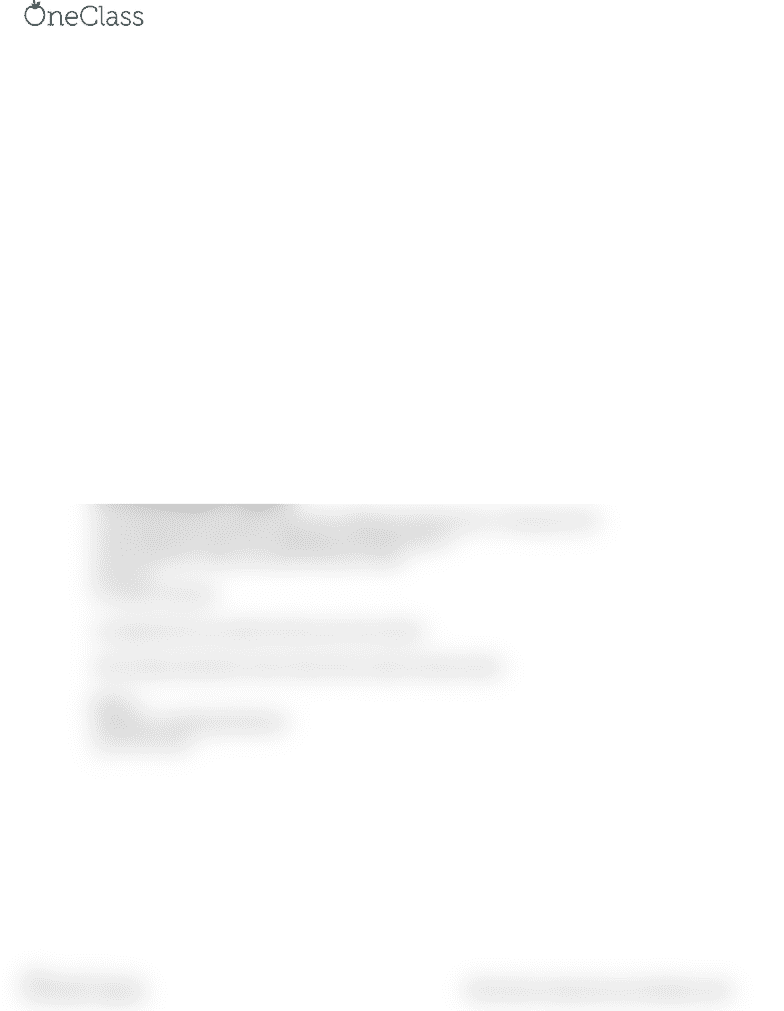# PSYC 3000 Lecture Notes - Lecture 2: Sampling Distribution, Analysis Of Variance

29 views1 pages
School
Department
CoursePSYC 3000
JAN 12
Contrast
-post hoc anylysis. Didn’t decide ahead of time make small amount of comparisons. So need to compare
every t tests.
-if you cant figure out what tests to do ahead of time then you cant discriminate which t tests you do
during post hoc. You must do them all.
-you can however before you gather your data which t test you will compare. This choice will not be
made for statistical reasons. It will be made due to prior studies or common knowledge which will allow
interesting questions to be answered. This will decrease the amount of tests and increase power.
-for example in the Eysenck data. The count and rhyme group can be compared to group 3,4&5.
-In assignment 4 you need to find what the interesting questions are. Explain a reason for every t test
you conduct.
-a contrast is a sum of means from experiments each multiplied by a coefficient
-the coefficient is determined by the number of groups within each group. (ex: if two groups are
compared against three groups then it will be (½ + ½) – (1/3 + 1/3 + 1/3). This allows each group to be
weighted the same way.
-the coefficient always equals 0
-the contrast test is a t test. S pooled is the denominator.
-the design will always be balanced
-ai2 in the formula you need to square the coefficient (1/2 becomes ¼. 1/3 become 1/9.)
-review slides from week 14 for assignment. Especially slide 31
-once you get the t value, you need to find the p value.
-df= k(n-1)
-k= amount of groups
-in assignment four we need to do four test. One contrast.
-look at paper presented in class to learn how to report contrast results.
ANOVA
-depends on sampling distribution
-test of the mean
-
find more resources at oneclass.com
find more resources at oneclass.com
Unlock document

This preview shows half of the first page of the document.
Unlock all 1 pages and 3 million more documents.

Already have an account? Log in

# Get access

Grade+
\$10 USD/m
Billed \$120 USD annually
Homework Help
Class Notes
Textbook Notes
40 Verified Answers
Study Guides
1 Booster Class
Class+
\$8 USD/m
Billed \$96 USD annually
Homework Help
Class Notes
Textbook Notes
30 Verified Answers
Study Guides
1 Booster Class